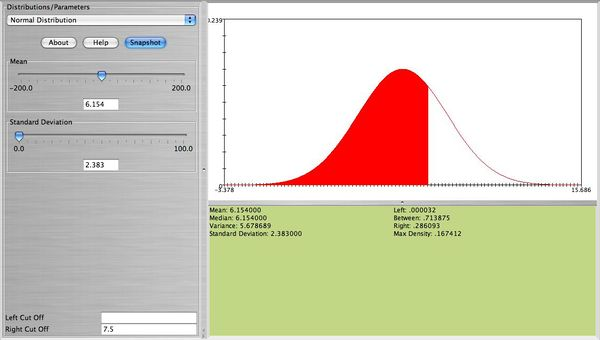# Difference between revisions of "AP Statistics Curriculum 2007 Limits Norm2Bin"

## General Advance-Placement (AP) Statistics Curriculum - Normal Distribution as Approximation to Binomial Distribution

### Normal Approximation to Binomial Distribution

Suppose $$Y\sim Binomial(n, p)$$ and $$Y=Y_1+ Y_2+ Y_3+\cdots+ Y_n$$, where $$Y_k~Bernoulli(p)$$ , $$E(Y_k)=p$$ & $$Var(Y_k)=p(1-p)$$. Then $$E(Y)=np$$, $$Var(Y)=np(1-p)$$ and $$SD(Y)= \sqrt{np(1-p)}$$. If we use the Normal Standardization formula for Y we get $$Z={Y-np\over \sqrt{np(1-p)}}$$.

By CLT, $$Z \sim N(0, 1)$$ and $$Y \sim N [\mu=np, \sigma^2={np(1-p)}]$$.

• Note: Normal approximation to Binomial is reasonable when p and (1-p) are NOT too small relative to n:

$np\geq 10$ $n(1-p)> 10$

### Example

The Roulette Game has 38 slots: 18 red, 18 black and 2 neutral. Suppose we play 100 games betting on red each time. Would observing 58 wins in the 100 games be considered atypical (suspicious)?

To answer this question, we need to compute the probability $$P(Y\geq58)$$, where $$Y\sim Binomial(100, 0.47)$$, as P(Win)=P(Red outcome) = 18/38=0.47.

Since $$np=47 \geq 10$$ and $$n(1-p)=53>10$$, Normal approximation is justified. Let $$Z={(Y-np)\over \sqrt{np(1-p)}} ={(58-100*0.47)\over \sqrt{100*0.47*0.53}}=2.2$$. Thus we can approximate $$P(Y\geq 58) \approx P(Z\geq 2.2) = 0.0139$$. The last equation can be directly computed using the SOCR Normal Distribution Calculator. You can also use the SOCR Binomial Distribution Calculator to compute the exact probability $$P(Y\geq 58) = 0.0177$$.

So why is the Normal approximation to Binomial distribution necessary in practice?

Binomial approximation by Normal distribution is useful when no access to the online SOCR resources is available (but we can use printed version of the Normal Table or when N is large and binomial probabilities are difficult to compute precisely!

### Activities

Graph and comment on the shape of binomial with $$n=20, p=0.1$$ and $$n=20, p=0.9$$. Now, keep $$n=20$$ but change $$p=0.45$$. What do you observe now? How about when $$n=80, p=0.1$$. See the four figures below.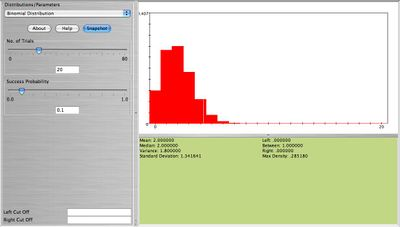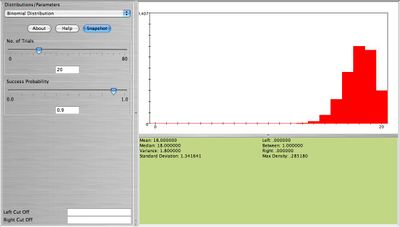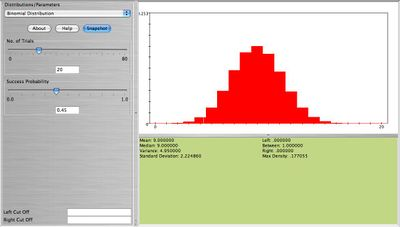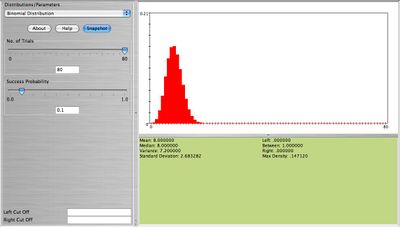What is your conclusion on the shape of the Binomial distribution in relation to its parameters $$n, p$$? Clearly when $$n$$ is large and $$p$$ small or large the result is a bell-shaped distribution. When $$n$$ is small (10-20) we still get approximately a bell-shaped distribution as long as $$p \approx 0.5$$. Because of this feature of the Binomial distribution we can approximate Binomial distributions using the normal distribution when the above requirements hold. Here is one example: Eighty cards are drawn with replacement from the standard 52-card deck. Find the exact probability that at least 8 aces are obtained. This can be computed using the formula $$P(X \ge 8)=\sum_{x=8}^{80}(\frac{4}{52})^x (\frac{48}{52})^{80-x}=0.2725$$.

Much easier we can use SOCR to compute this probability (see figure below).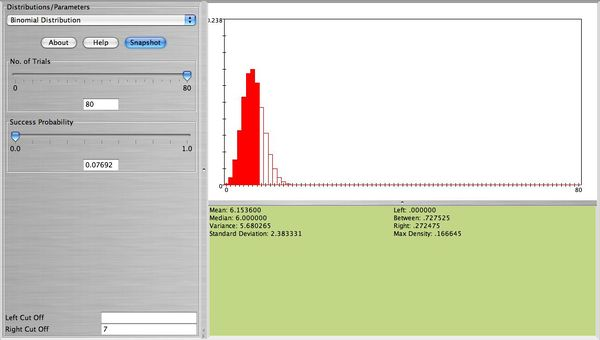But we can also approximate this probability using the normal distribution. We will need the mean and the standard deviation of this normal distribution. These are $$\mu=np=80\frac{4}{52}=6.154$$ and $$\sigma=\sqrt{80 \frac{4}{52}\frac{48}{52}}=2.383.$$ Of course this can be obtained directly from the SOCR Binomial applet. Now, all you need to do is to select the SOCR normal distribution applet and enter for the mean 6.154, and for the standard deviation 2.383. To obtain the desire probability in the right cut-off box enter 7.5 (using the continuity correction for better approximation). The approximate probability is $$P(X \ge 8) \approx 0.2861$$ (see figure below).